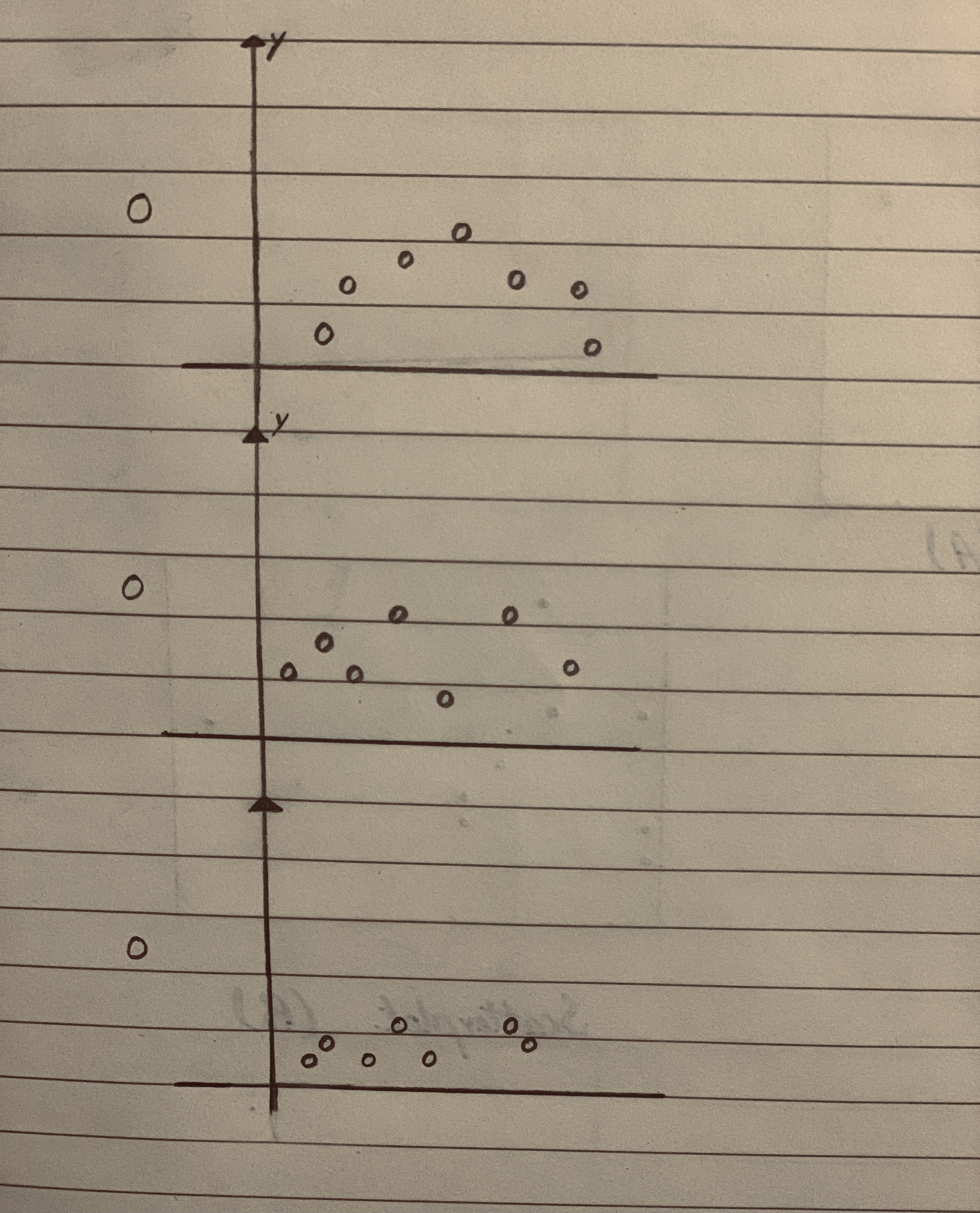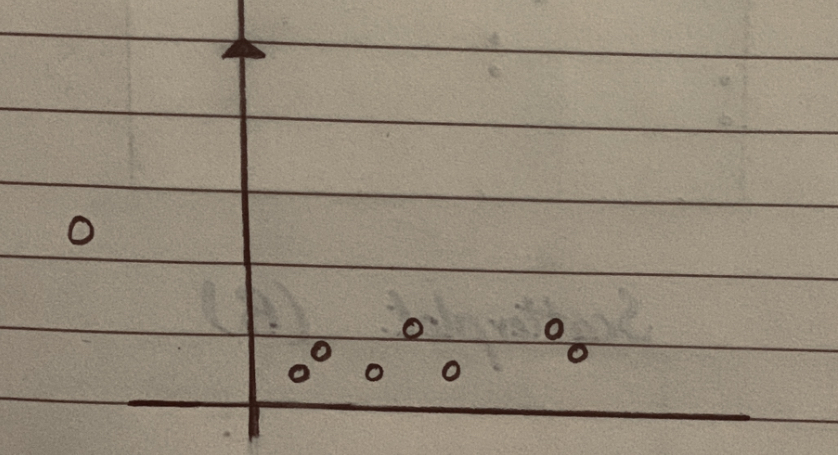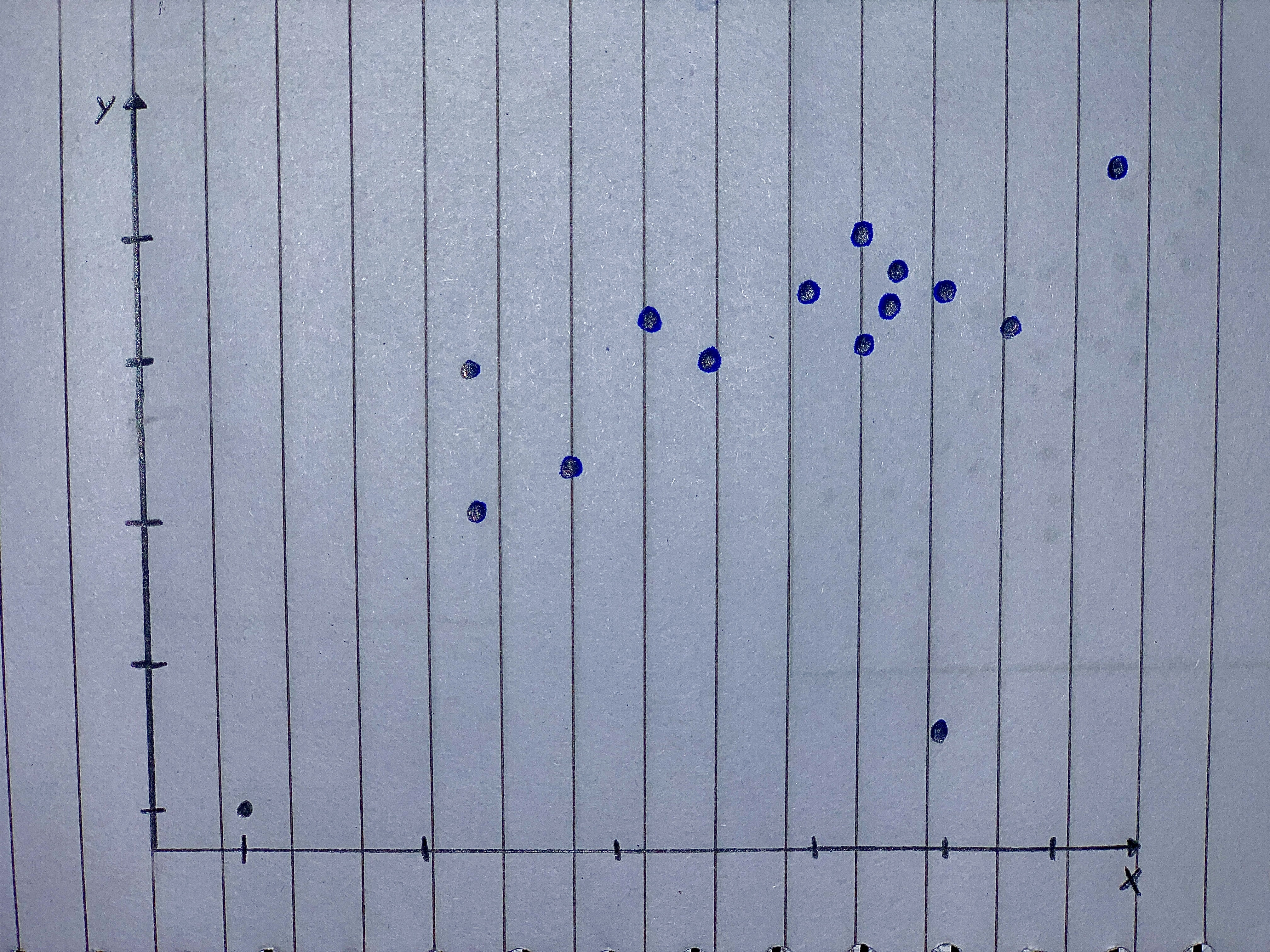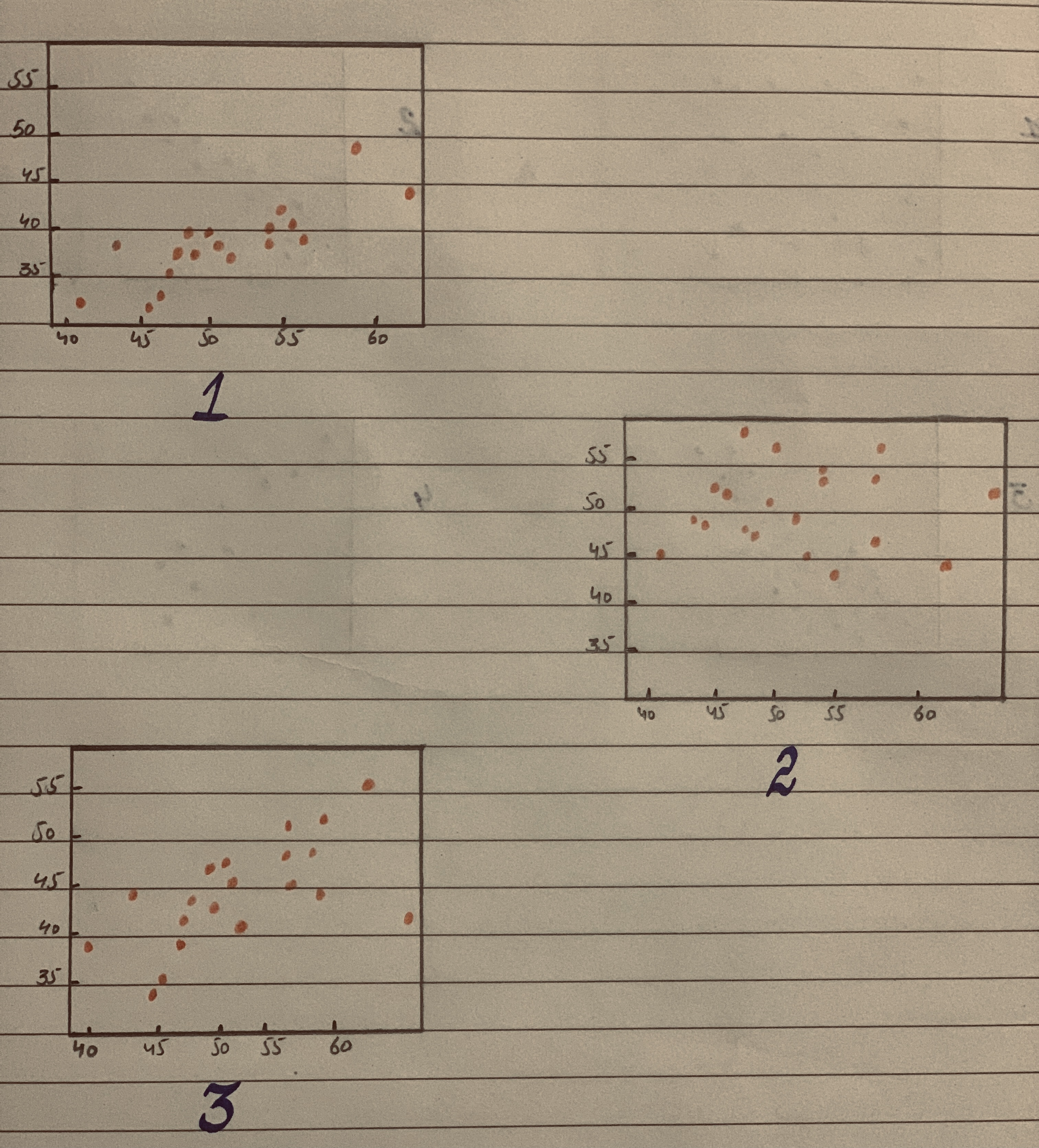Ask question

# Which of these shows the strongest correlation?# Which of these shows the strongest correlation?

Question
Scatterplotsasked 2020-11-07

Which of these shows the strongest correlation?## Answers (1)2020-11-08
A linear relationship can be best described by a straight line. In the scatterplot, if a straight line is drawn, all the data points lie on the straight line or around the straight line.
As compare to other scatterplots, scatterplot 3 indicates that most of the data points form a straight line or lies close to the straight line, hence this scatterplot represents the strongest linear correlation.### Relevant Questionsasked 2021-01-08

Make a scatterplot of the data and graph the function $$\displaystyle{f{{\left({x}\right)}}}=\ -{8}{x}^{{{2}}}\ +\ {95}{x}\ +\ {745}.$$ Make a residual plot and describe how well the function fits the data. $$\begin{array}{|c|c|} \hline \text{Price Increase} & 0 & 1 & 2 & 3 & 4 \\ \hline \text{Sales} & 730 & 850 & 930 & 951 & 1010 \\ \hline \end{array}$$asked 2021-02-25

a. Make a scatterplot for the data in the table below.
Height and Weight of Football Players
$$\begin{array}{|c|c|}\hline \text{Height (in.):} & 77 & 75 & 76 & 70 & 70 & 73 & 74 & 74 & 73 \\ \hline \text{Weight (lb):} & 230 & 220 & 212 & 190 & 201 & 245 & 218 & 260 & 196 \\ \hline \end{array}$$

b. Which display - the table or the scatter plot - do you think is a more appropriate display of the data? Explain your reasoning.asked 2021-02-03

The following data on = soil depth (in centimeters) and y = percentage of montmorillonite in the soil were taken from a scatterplot in the paper "Ancient Maya Drained Field Agriculture: Its Possible Application Today in the New River Floodplain, Belize, C.A." (Agricultural Ecosystems and Environment : 67-84):
a. Draw a scatterplot of y versus x.
b. The equation of the least-squares line is 0.45x. Draw this line on your scatterplot. Do there appear to be any large residuals?
c. Compute the residuals, and construct a residual plot. Are there any unusual features in the plot?
$$\begin{array}{|c|c|}\hline x & 40 & 50 & 60 & 70 & 80 & 90 & 100 \\ \hline y & 58 & 34 & 32 & 30 & 28 & 27 & 22 \\ \hline \end{array}$$
$$\displaystyle{\left[\hat{{{y}}}={64.50}\right]}$$.asked 2021-02-19

Make a scatterplot for each set of data. Tell whether the data show a linear association or a nonlinear association.
$$(1,\ 2),\ (7,\ 9.5),\ (4,\ 7),\ (2,\ 4.2),\ (6,\ 8.25),\ (3,\ 5.8),\ (5,\ 8),\ (8,\ 10),\ (0,\ 0)$$asked 2021-03-06

Make a scatterplot for the data.
Height and Weight of Females
$$\begin{array}{|c|c|}\hline \text{Height (in.):} & 58 & 60 & 62 & 64 & 65 & 66 & 68 & 70 & 72 \\ \hline \text{Weight (lb):} & 115 & 120 & 125 & 133 & 136 & 115 & 146 & 153 & 159 \\ \hline \end{array}$$asked 2021-01-04

State the slope and y-intercept of the equation. $$5x + 4y = 20$$asked 2021-01-31

Make a scatterplot for each set of data.
$$\begin{array}{|c|c|}\hline \text{Hits:} & 7 & 8 & 4 & 11 & 8 & 2 & 5 & 9 & 1 & 4 \\ \hline \text{Runs:} & 3 & 2 & 2 & 7 & 4 & 2 & 1 & 3 & 0 & 1 \\ \hline \end{array}$$asked 2021-01-13

The accompanying data on y = normalized energy $$\displaystyle{\left[{\left(\frac{{J}}{{m}^{{2}}}\right)}\right]}$$ and x = intraocular pressure (mmHg) appeared in a scatterplot in the article “Evaluating the Risk of Eye Injuries: Intraocular Pressure During High Speed Projectile Impacts” (Current Eye Research, 2012: 43-49), an estimated regression function was superimposed on the plot.
$$\begin{array}{|c|c|}\hline x & 2761 & 19764 & 25713 & 3980 & 12782 & 19008 & 19028 & 14397 & 9606 & 3905 & 25731 \\ \hline y & 1553 & 14999 & 32813 & 1667 & 8741 & 16526 & 26770 & 16526 & 9868 & 6640 & 1220 & 30730 \\ \hline \end{array}$$
Here is Minitab output from fitting the simple linear regression model. Does the model appear to specify a useful relationship between the two variables?
$$\begin{array}{|c|c|}\hline \text{Predictor Coef SE Coef T P Constant} & -5090 & 2257 & -2.26 & 0.048 \\ \hline \text{Pressure} & 1.2912 & 0.1347 & 9.59 & 0.000 \\ \hline \end{array}$$
$$[S=3679.36, R-Sq = 90.2\%, R-Sq(adj)=89.2\% ]$$.asked 2020-11-29

Straight Lines Graph the straight lines in Exercises 1–3.
Then find the change in y for a one-unit change in x, find the point at which the line crosses the y-axis, and calculate the value of y when x 52.5.
1. $$y x\ 5\ 1\ 2.0\ 0.5$$
2. $$y x\ 5\ 1\ 40\ 36.2$$
3. $$y x\ 5\ 25\ 6$$
Scatterplots For the scatterplots in Exercises 5 describe the pattern that you see. How strong is the pattern? Do you see any outliers or clusters?asked 2020-12-07

The scatterplots below display three bivariate data sets. The correlation coefficients for these data sets are 0.03, 0.68, and 0.89. Which scatter plot corresponds to the data set with r = .03?...